# C语言 自增和自减

+ 把两个操作数相加 A + B 将得到 30

* 把两个操作数相乘 A * B 将得到 200
/ 分子除以分母 B / A 将得到 2
% 取模运算符，整除后的余数 B % A 将得到 0
++ 自增运算符，整数值增加 1 A++ 将得到 11

• 前置
• 变量自增（减）1
• 取变量值
• 后置
• 取变量值
• 变量自增（减）1

## 示例：自增和自减运算符

#include <stdio.h>

int main()
{
int i = 0;
int r = 0;

r = (i++) + (i++) + (i++);

printf("i = %d\n", i);
printf("r = %d\n", r);

r = (++i) + (++i) + (++i);

printf("i = %d\n", i);
printf("r = %d\n", r);

return 0;
}


gcc输出结果：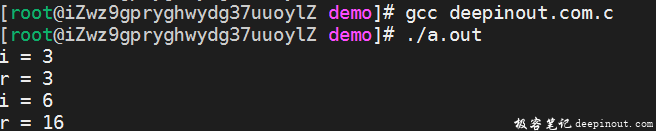VC10.0输出结果：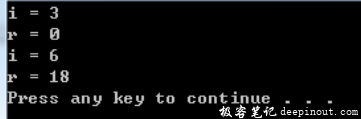++, — 操作符使用分析

• C语言中只规定了++和–对应指令的相对执行次序
• ++ 和 — 对应的汇编指令不一定连续运行，如下面的汇编指令
•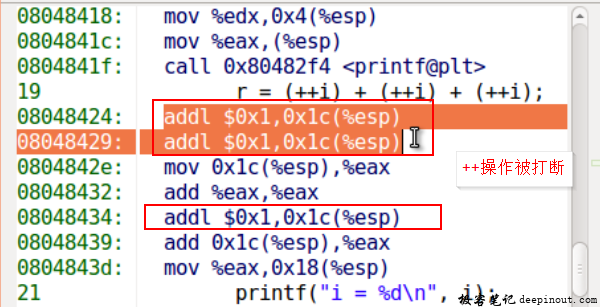• 在混合运算中， ++ 和 — 的汇编指令可能被打断执行

## 自增和自减表达式阅读技巧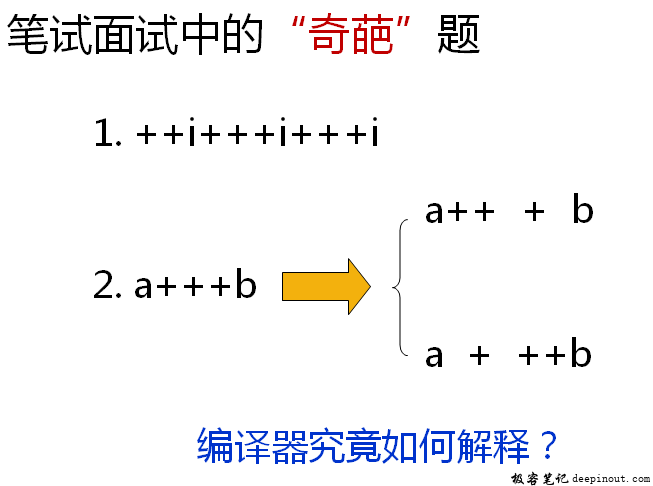• 编译器处理的每个符合应该尽可能多的包含字符
• 编译器从左向右的顺序一个一个尽可能多的读入字符
• 当读入的字符不可能和已读入的字符组成合法符号为止

### 贪心法阅读示例

#include <stdio.h>

int main()
{
int i = 0;
//int j = ++i+++i+++i; //编译出错， 通过贪心法，编译器先读到 ++i++， 变成 1++，显然有问题

int a = 1;
int b = 4;
int c = a+++b; // a+++b; 1 + 4 ====>5   a ==> 2

int* p = &a;

b = b / *p;

printf("i = %d\n", i);
//printf("j = %d\n", j);
printf("a = %d\n", a);
printf("b = %d\n", b);
printf("c = %d\n", c);

return 0;
}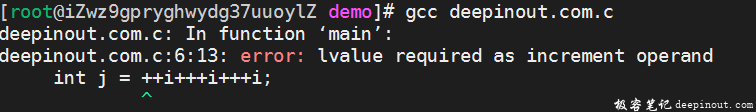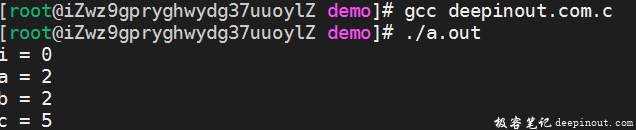## 总结

• 自增和自减操作符在混合运算中的行为可能不同
• 编译器通过贪心法处理表达式中的子表达式
• 空格可以作为C语言中一个完整符合的休止符
• 编译器读入空格后立即对之前读入的符号进行处理

• 微信订阅
• 回顶